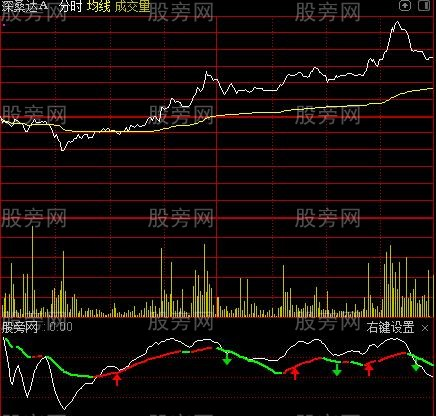# 通达信小神仙秘密线分时指标公式

http://www.gupang.com/202002/49972.html

HJ_1:=MA(LOW,24)*1.09;
HJ_2:=CROSS(HJ_1,OPEN);
HJ_3:=MA((LOW+HIGH+CLOSE+OPEN)/4,25)*1.23;
HJ_4:=CROSS(HJ_3,OPEN);
HJ_5:=EMA(LOW,25);
HJ_6:=CROSS(CLOSE,HJ_5);
HJ_7:=REF(COUNT(REF(EMA(CLOSE,5)/REF(EMA(CLOSE,5),1)<1,1) AND EMA(CLOSE,5)/REF(EMA(CLOSE,5),1)>1,11)>=1,3) AND REF(EMA(CLOSE,5)/REF(EMA(CLOSE,5),1)>0.987,1) AND COUNT(OPEN<CLOSE,7)/7>5/10 AND REF(COUNT(CLOSE/REF(CLOSE,1)>1.08 OR CLOSE/REF(CLOSE,2)>1.08 AND CLOSE/OPEN>1.038,25)>=1,3) AND COUNT(CLOSE>OPEN,2)=2 AND CLOSE>REF(CLOSE,1) AND (LOW<MA(CLOSE,5) OR REF(LOW<MA(CLOSE,5) AND CLOSE/REF(CLOSE,1)>1.095 AND HIGH=CLOSE,1) OR (COUNT(LOW>MA(CLOSE,5),3)=3 AND OPEN/MA(CLOSE,5)<1.015)) AND COUNT(REF(CLOSE<REF(CLOSE,1),1) AND CLOSE>OPEN,9)>=2 AND REF(COUNT(CLOSE>OPEN AND HIGH>MA(CLOSE,5) AND CLOSE/REF(CLOSE,1)<1.095,5)>=1,1) AND CLOSE>MA(CLOSE,5);
HJ_8:=REF(COUNT(HJ_5,2)>0,3) AND (OPEN<REF(OPEN,1) OR LOW<REF(LOW,1) OR VOL>REF(VOL,1));
HJ_9:=REF(COUNT(CLOSE/REF(CLOSE,1)>1.095 AND HIGH=CLOSE,5)>=1 AND OPEN>MA(CLOSE,5),3) AND REF(COUNT(HIGH/LOW>1.095 AND CLOSE<HIGH,2)>=1,2) AND REF(CLOSE/REF(CLOSE,1)<0.907 AND LOW/CLOSE>0.996 AND COUNT(HIGH>OPEN,2)>=1 AND COUNT(VOL>REF(VOL,1),3)>=1,1) AND REF(CLOSE,1)/LOW>1.04 AND CLOSE/LOW>1.02;
HJ_10:=HJ_9 AND OPEN/LOW<1.033 AND VOL<HHV(VOL,3) OR (REF(COUNT(HJ_9,2)>=1,3) AND REF(CLOSE/REF(CLOSE,1)<0.911,1) AND OPEN/REF(CLOSE,1)>0.998 AND OPEN/REF(CLOSE,1)<1.033) OR (REF(HJ_9,4) AND REF(COUNT(CLOSE/REF(CLOSE,1)<0.907,2)>=1,1) AND (REF(CLOSE,1)/LOW>1.086 AND CLOSE/LOW>1.02 OR (OPEN/REF(CLOSE,1)>0.998 AND OPEN/REF(CLOSE,1)<1.033)));
HJ_11:=HJ_10 AND OPEN/LOW<1.033 AND OPEN/CLOSE<1.033 OR (REF(COUNT(HJ_10,2)>=1,3) AND REF(CLOSE/REF(CLOSE,1)<0.911 OR (OPEN<REF(CLOSE,1) AND CLOSE/REF(CLOSE,1)<0.95),1) AND OPEN/REF(CLOSE,1)>0.998 AND OPEN/REF(CLOSE,1)<1.033 AND OPEN/CLOSE<1.033) OR (REF(HJ_9,4) AND REF(COUNT(CLOSE/REF(CLOSE,1)<0.907,2)>=1,1) AND OPEN/CLOSE<1.033 AND (REF(CLOSE,1)/LOW>1.086 AND CLOSE/LOW>1.02 OR (OPEN/REF(CLOSE,1)>0.998 AND OPEN/REF(CLOSE,1)<1.033)));
HJ_12:=1000*CLOSE/INDEXC;
HJ_13:=1000*OPEN/INDEXO;
HJ_14:=1000*LOW/INDEXL;
HJ_15:=100*EMA(EMA(CLOSE-REF(CLOSE,1),6),6)/EMA(EMA(ABS(CLOSE-REF(CLOSE,1)),6),6);
HJ_16:=LLV(HJ_15,2)=LLV(HJ_15,7) AND COUNT(HJ_15<0,2) AND CROSS(HJ_15,MA(HJ_15,2));
HJ_17:=CLOSE/REF(CLOSE,1)>=1.08 OR CLOSE/OPEN>1.06 AND (HJ_16 AND NOT(HJ_2) AND NOT(REF(HJ_2,4)) AND (NOT(REF(HJ_6 AND HJ_16,3)) AND NOT(REF(HJ_16 AND REF(CLOSE>OPEN,1),3))) OR (REF(HJ_16,4) AND CLOSE/REF(CLOSE,1)>1.095 AND HIGH=CLOSE AND REF(COUNT(HJ_12<HJ_13,5)>=1,1) AND REF(HJ_12>HJ_13,3)) OR (REF(HJ_11 OR REF(HJ_11,4),1) AND CLOSE/REF(CLOSE,1)>1.095 AND HIGH=CLOSE)) AND LOW>REF(LOW,1) AND LOW/MA(CLOSE,5)<1.025;
HJ1:=REF(COUNT(HJ_17,3)>=1,2) AND REF(CLOSE>=OPEN AND REF(CLOSE/REF(CLOSE,1)>=1.08 OR CLOSE/OPEN>1.067 AND CLOSE/REF(CLOSE,1)>=1.078,1) AND HIGH/CLOSE>1.025 AND HIGH/CLOSE>OPEN/LOW,1) AND CLOSE>=REF(OPEN,1) AND OPEN/REF(CLOSE,1)<1.03 AND COUNT(LOW/MA(CLOSE,5)<1.027,2)>=1;
HJ2:=(COUNT(HJ_12>HJ_13,3)=3 OR (COUNT(HJ_12>HJ_13,3)>=2 AND REF(HJ_11,3) AND REF(CLOSE/REF(CLOSE,1)>1.095 AND HIGH=CLOSE,2)));
HJ_18:=HJ1 AND (HJ_13/HJ_14>REF(HJ_13/HJ_14,2) OR REF(HJ_16,5) AND REF(REF(REF(COUNT(HJ_12>HJ_13,4)>=1,1) AND HJ_12<HJ_13,1) OR HJ_12>HJ_13,7) AND REF(HIGH/1.03<REF(HHV(HIGH,3),1),2) OR (REF(COUNT(HJ_12>HJ_13,2)=2,7) AND REF(COUNT(HJ_12<HJ_13,3)>=1,6) AND REF(HJ_12<HJ_13,4) AND REF(HIGH,2)>REF(HIGH,3)) OR REF(COUNT(HJ_12>HJ_13,2)=2 AND (REF(HJ_12<HJ_13,7) OR REF(HJ_12>HJ_13,2)),3) OR REF(COUNT(HJ_12>HJ_13,3)=3 AND REF(HJ_12>HJ_13,6),4) OR (REF(COUNT(HJ_12>HJ_13,2)=2,1) AND HJ_7 AND REF(HJ_12>HJ_13,6) AND REF(COUNT(HJ_12<HJ_13,3)>=1,7) AND REF(COUNT(HJ_12<HJ_13,3)>=2,3))) AND HJ_12/HJ_13>REF(HJ_12/HJ_13,1) AND CLOSE/OPEN>REF(CLOSE/OPEN,1) AND HJ2;
HJ_19:=CLOSE/REF(CLOSE,1)>=1.095 AND HIGH=CLOSE AND REF(CLOSE/OPEN<1.05,1) AND (COUNT(HJ_7,3)>=1 OR REF(HJ_4,1)) AND COUNT(LOW<REF(LOW,1),2)>=1 AND LOW<MA(CLOSE,5) AND NOT(REF(COUNT(CLOSE/REF(CLOSE,1)<0.923,3)>=1,1));
HJ_20:=REF(HJ_19,2) AND REF(CLOSE<=OPEN AND (HIGH/OPEN>1.043 AND REF(CLOSE>OPEN,4) OR (HIGH/CLOSE>1.089 AND OPEN/CLOSE>1.039 AND HIGH>OPEN AND REF(CLOSE/REF(CLOSE,1)>1.095 AND HIGH=CLOSE,1))),1) AND OPEN/LOW<1.029 AND OPEN/REF(LOW,3)>0.977 AND OPEN/REF(OPEN,2)<1.077 AND CLOSE/REF(CLOSE,1)>0.989 AND OPEN/REF(CLOSE,1)<1 AND COUNT(LOW<MA(CLOSE,5),2)>=1 AND REF(HJ_12<HJ_13,1) AND COUNT(HJ_12>HJ_13,3)>=2;
HJ_21:=MA(LOW,24)*1.09;
HJ_22:=CROSS(HJ_21,OPEN);
HJ_23:=MA((LOW+HIGH+CLOSE+OPEN)/4,25)*1.23;
HJ_24:=CROSS(HJ_23,OPEN);
HJ_25:=EMA(LOW,25);
HJ_26:=CROSS(CLOSE,HJ_25);
HJ_27:=REF(COUNT(REF(EMA(CLOSE,5)/REF(EMA(CLOSE,5),1)<1,1) AND EMA(CLOSE,5)/REF(EMA(CLOSE,5),1)>1,11)>=1,3) AND REF(EMA(CLOSE,5)/REF(EMA(CLOSE,5),1)>0.987,1) AND COUNT(OPEN<CLOSE,7)/7>5/10 AND REF(COUNT(CLOSE/REF(CLOSE,1)>1.08 OR CLOSE/REF(CLOSE,2)>1.08 AND CLOSE/OPEN>1.038,25)>=1,3) AND COUNT(CLOSE>OPEN,2)=2 AND CLOSE>REF(CLOSE,1) AND (LOW<MA(CLOSE,5) OR REF(LOW<MA(CLOSE,5) AND CLOSE/REF(CLOSE,1)>1.095 AND HIGH=CLOSE,1) OR (COUNT(LOW>MA(CLOSE,5),3)=3 AND OPEN/MA(CLOSE,5)<1.015)) AND COUNT(REF(CLOSE<REF(CLOSE,1),1) AND CLOSE>OPEN,9)>=2 AND REF(COUNT(CLOSE>OPEN AND HIGH>MA(CLOSE,5) AND CLOSE/REF(CLOSE,1)<1.095,5)>=1,1) AND CLOSE>MA(CLOSE,5);
HJ_28:=REF(COUNT(CLOSE/REF(CLOSE,1)>1.095 AND HIGH=CLOSE,5)>=1 AND OPEN>MA(CLOSE,5),3) AND REF(COUNT(HIGH/LOW>1.095 AND CLOSE<HIGH,2)>=1,2) AND REF(CLOSE/REF(CLOSE,1)<0.907 AND LOW/CLOSE>0.996 AND COUNT(HIGH>OPEN,2)>=1 AND COUNT(VOL>REF(VOL,1),3)>=1,1) AND REF(CLOSE,1)/LOW>1.04 AND CLOSE/LOW>1.02;
HJ_29:=HJ_28 AND OPEN/LOW<1.033 AND VOL<HHV(VOL,3) OR (REF(COUNT(HJ_28,2)>=1,3) AND REF(CLOSE/REF(CLOSE,1)<0.911,1) AND OPEN/REF(CLOSE,1)>0.998 AND OPEN/REF(CLOSE,1)<1.033) OR (REF(HJ_28,4) AND REF(COUNT(CLOSE/REF(CLOSE,1)<0.907,2)>=1,1) AND (REF(CLOSE,1)/LOW>1.086 AND CLOSE/LOW>1.02 OR (OPEN/REF(CLOSE,1)>0.998 AND OPEN/REF(CLOSE,1)<1.033)));
HJ_30:=SUM(IF(CLOSE>REF(CLOSE,1) AND VOL>4000,VOL/1000,0),0);
HJ_31:=SUM(IF(CLOSE<REF(CLOSE,1) AND VOL>4000,VOL/1000,0),0);
NOTEXT1:IF(HJ_30,HJ_30,0),COLORCC9932,LINETHICK4;
NOTEXT2:IF(HJ_31,HJ_31,0),COLORGREEN,LINETHICK2;
HJ_32:=(2*CLOSE+HIGH+LOW)/4;
HJ_33:=LLV(LOW,94);
HJ_34:=HHV(HIGH,89);
HJ_35:=EMA((CLOSE-HJ_33)/(HJ_34-HJ_33)*100,8);
HJ_36:=EMA(0.667*REF(HJ_35,1)+0.333*HJ_35,10);
HJ_37:=30;
HJ_38:=15;
HJ_39:=(CLOSE-LLV(LOW,40))/(HHV(HIGH,40)-LLV(LOW,40))*100;
HJ_40:=SMA(HJ_39,HJ_37,1);
HJ_41:=SMA(HJ_40,HJ_38,1);
HJ_42:=HJ_40;
HJ_43:=IF(HJ_42>REF(HJ_42,1),HJ_42,DRAWNULL);
HJ_44:=IF(HJ_42<REF(HJ_42,1),HJ_42,DRAWNULL);
DRAWICON(CROSS(HJ_40,HJ_41),HJ_40,1);
DRAWICON(CROSS(HJ_41,HJ_40),HJ_40,2);
HJ_45:=HJ_29 AND OPEN/LOW<1.033 AND OPEN/CLOSE<1.033 OR (REF(COUNT(HJ_29,2)>=1,3) AND REF(CLOSE/REF(CLOSE,1)<0.911 OR (OPEN<REF(CLOSE,1) AND CLOSE/REF(CLOSE,1)<0.95),1) AND OPEN/REF(CLOSE,1)>0.998 AND OPEN/REF(CLOSE,1)<1.033 AND OPEN/CLOSE<1.033) OR (REF(HJ_28,4) AND REF(COUNT(CLOSE/REF(CLOSE,1)<0.907,2)>=1,1) AND OPEN/CLOSE<1.033 AND (REF(CLOSE,1)/LOW>1.086 AND CLOSE/LOW>1.02 OR (OPEN/REF(CLOSE,1)>0.998 AND OPEN/REF(CLOSE,1)<1.033)));
HJ_46:=1000*CLOSE/INDEXC;
HJ_47:=1000*OPEN/INDEXO;
HJ_48:=1000*LOW/INDEXL;
HJ_49:=100*EMA(EMA(CLOSE-REF(CLOSE,1),6),6)/EMA(EMA(ABS(CLOSE-REF(CLOSE,1)),6),6);
HJ_50:=LLV(HJ_49,2)=LLV(HJ_49,7) AND COUNT(HJ_49<0,2) AND CROSS(HJ_49,MA(HJ_49,2));
HJ_51:=MA(CLOSE,5)<MA(CLOSE,10) AND LOW<MA(CLOSE,5) AND HIGH/OPEN>1.07 AND OPEN=LOW AND CLOSE>OPEN AND OPEN/REF(CLOSE,1)<0.95 AND HIGH/CLOSE>1.043 AND (VOL>REF(HHV(VOL,800),1) OR REF(CLOSE>LOW,1));
HJ_52:=CLOSE/REF(CLOSE,1)>=1.08 OR CLOSE/OPEN>1.06 AND (HJ_50 AND NOT(HJ_22) AND (NOT(REF(HJ_26 AND HJ_50,3)) AND NOT(REF(HJ_50 AND REF(CLOSE>OPEN,1),3))) OR (REF(HJ_50,4) AND CLOSE/REF(CLOSE,1)>1.095 AND HIGH=CLOSE AND REF(COUNT(HJ_46<HJ_47,5)>=1,1) AND REF(HJ_46>HJ_47,3)) OR (REF(HJ_45 OR REF(HJ_45,4),1) AND CLOSE/REF(CLOSE,1)>1.095 AND HIGH=CLOSE)) AND LOW>REF(LOW,1) AND LOW/MA(CLOSE,5)<1.025;
HJ_53:=REF(COUNT(HJ_52,4)>=1 AND CLOSE>OPEN AND REF(COUNT(CLOSE/REF(CLOSE,1)>1.095 AND HIGH=CLOSE,3)=3,1),3) AND REF(OPEN<REF(CLOSE,1) AND OPEN/CLOSE>1.01 AND HIGH/CLOSE>1.025 AND HIGH/CLOSE>OPEN/LOW,2) AND REF(CLOSE<OPEN AND HIGH/OPEN>CLOSE/LOW AND HIGH/OPEN>OPEN/CLOSE OR (CLOSE>OPEN AND HIGH/CLOSE>CLOSE/OPEN),1) AND (REF(CLOSE,1)<REF(OPEN,2) AND REF(CLOSE/OPEN<1,2) OR (REF(CLOSE,1)<REF(CLOSE,2) AND REF(CLOSE/OPEN>1,2))) AND (CLOSE/REF(CLOSE,1)>1.041 OR CLOSE/OPEN>1.039) AND REF(CLOSE/OPEN<1.051 OR CLOSE/REF(CLOSE,1)<1.051,1) AND (REF(LOW,1)<REF(LOW,2) OR REF(LOW,2)<REF(LOW,3) AND COUNT(CLOSE/REF(CLOSE,1)<0.9672 OR CLOSE/OPEN<0.9672,8)>=1 AND CLOSE>ACD AND (COUNT(CLOSE>ACD,2)>=1 OR REF(CLOSE/OPEN<1.051,1)) AND REF(COUNT(CLOSE/OPEN>1.0595,7)>=1 OR COUNT(CLOSE/REF(CLOSE,1)>1.0595,7)>=1,3) AND REF(CLOSE/OPEN<1.0097 OR CLOSE/REF(CLOSE,1)<1.0097,1) AND CLOSE>REF(CLOSE,1));
HJ_54:=REF(COUNT(HJ_52,3)>=1 AND CLOSE>OPEN AND REF(COUNT(CLOSE/REF(CLOSE,1)>1.095 AND HIGH=CLOSE,2)=2,1),5) AND REF(OPEN<REF(CLOSE,1) AND OPEN/CLOSE>1.01 AND HIGH/CLOSE>1 AND HIGH/CLOSE>OPEN/LOW,4) AND REF(HIGH/CLOSE>CLOSE/OPEN AND REF(CLOSE<REF(CLOSE,1),1),1) AND (CLOSE/REF(CLOSE,1)>1.041 OR CLOSE/OPEN>1.039) AND REF(CLOSE/OPEN<1.058 OR CLOSE/REF(CLOSE,1)<1.058,1) AND (REF(LOW,1)<REF(LOW,2) OR REF(LOW,2)<REF(LOW,3) AND COUNT(CLOSE/REF(CLOSE,1)<0.967 OR CLOSE/OPEN<0.967,8)>=1 AND CLOSE>ACD AND (COUNT(CLOSE>ACD,2)>=1 OR REF(CLOSE/OPEN<1.05,1)) AND REF(COUNT(CLOSE/OPEN>1.0595,7)>=1 OR COUNT(CLOSE/REF(CLOSE,1)>1.0595,7)>=1,3) AND (REF(CLOSE/OPEN<1,2) AND CLOSE>REF(CLOSE,2) OR (REF(CLOSE/OPEN>1,2) AND CLOSE>REF(OPEN,2))));
NOTEXT3:IF(HJ_35,HJ_35,0),COLORWHITE;
NOTEXT4:IF(HJ_43,HJ_43,0),COLORRED,LINETHICK2;
NOTEXT5:IF(HJ_44,HJ_44,0),COLORGREEN,LINETHICK2;
0,COLORGRAY,LINETHICK3;
HJ3:=REF(COUNT(HJ_52,3)>=1,2) AND REF(C>=O OR C/REF(C,1)<0.95,1) AND REF(C>=O AND REF(C/REF(C,1)>=1.08 OR C/O>1.067 AND C/REF(C,1)>=1.0785,1) OR (REF(COUNT(C/REF(C,1)>1.095 AND H=C,2)=2,2) AND O<REF(C,1) AND O/C>1.01) AND H/C>1.025 AND (H/C>O/L OR (C>O AND REF(C/REF(C,1)>1.095 AND H=C AND HJ_50,1))),1);
HJ4:=(REF(HJ_51 AND HJ_47/REF(HJ_47,1)>0.9,1) AND HJ_47>REF(HJ_47,1) AND O/L<1.045) AND REF(C/O<1.06,1) AND C/O>REF(C/O,1);
HJ5:=C>=REF(O,1) AND O/REF(C,1)<1.03 AND COUNT(L/MA(C,5)<1.027,2)>=1;
HJ6:=REF(REF(REF(COUNT(HJ_46>HJ_47,4)>=1,1) AND HJ_46<HJ_47,1) OR HJ_46>HJ_47,7);
HJ7:=HJ_47/HJ_48>REF(HJ_47/HJ_48,2) OR REF(HJ_50,5) AND HJ6 AND REF(H/1.03<REF(HHV(H,3),1),2);
HJ8:=(REF(COUNT(HJ_46>HJ_47,2)=2,7) AND REF(COUNT(HJ_46<HJ_47,3)>=1,6) AND REF(HJ_46<HJ_47,4) AND REF(H,2)>REF(H,3));
HJ_55:=HJ3 AND HJ5 AND (L>REF(L,1) OR O/REF(C,2)<0.97 AND (HJ7) OR HJ8 OR REF(COUNT(HJ_46>HJ_47,2)=2 AND (REF(HJ_46<HJ_47,7) OR REF(HJ_46>HJ_47,2)),3) OR REF(COUNT(HJ_46>HJ_47,3)=3 AND REF(HJ_46>HJ_47,6),4) OR (REF(COUNT(HJ_46>HJ_47,2)=2,1) AND HJ_27 AND REF(HJ_46>HJ_47,6) AND REF(COUNT(HJ_46<HJ_47,3)>=1,7) AND REF(COUNT(HJ_46<HJ_47,3)>=2,3))) AND HJ_46/HJ_47>REF(HJ_46/HJ_47,1) AND (COUNT(HJ_46>HJ_47,3)=3 OR (COUNT(HJ_46>HJ_47,3)>=2 AND (REF(HJ_45,3) AND REF(C/REF(C,1)>1.095 AND H=C,2)) OR (REF(COUNT(C/REF(C,1)>1.095 AND H=C,2)=2,3) AND REF(C>O,2)))) OR HJ4;
HJ_56:=CLOSE/REF(CLOSE,1)>=1.095 AND HIGH=CLOSE AND REF(CLOSE/OPEN<1.05,1) AND (COUNT(HJ_27,3)>=1 OR REF(HJ_24,1)) AND COUNT(LOW<REF(LOW,1),2)>=1 AND LOW<MA(CLOSE,5) AND NOT(REF(COUNT(CLOSE/REF(CLOSE,1)<0.923,3)>=1,1));
HJ_57:=REF(HJ_56,2) AND REF(CLOSE<=OPEN,1) AND (REF(HIGH/OPEN>1.043 AND REF(CLOSE>OPEN,4) OR (HIGH/CLOSE>1.089 AND OPEN/CLOSE>1.039 AND HIGH>OPEN AND REF(CLOSE/REF(CLOSE,1)>1.095 AND HIGH=CLOSE,1)),1) AND OPEN/LOW<1.029 AND OPEN/REF(OPEN,2)<1.077 OR (REF(HIGH/OPEN>1.025 AND REF(CLOSE>OPEN,4) OR (HIGH/CLOSE>1.089 AND OPEN/CLOSE>1.039 AND HIGH>OPEN AND REF(CLOSE/REF(CLOSE,1)>1.095 AND HIGH=CLOSE,1)),1) AND OPEN/LOW<1.053 AND REF(CLOSE<OPEN AND CLOSE/LOW<1.02,3))) AND OPEN/REF(LOW,3)>0.977 AND CLOSE/REF(CLOSE,1)>0.989 AND OPEN/REF(CLOSE,1)<1 AND COUNT(LOW<MA(CLOSE,5),2)>=1 AND REF(HJ_46<HJ_47,1) AND COUNT(HJ_46>HJ_47,3)>=2;
HJ_58:=REF(CLOSE,1)>REF(CLOSE,2) AND CLOSE/REF(CLOSE,1)<1.05 AND REF(CLOSE,1)/REF(OPEN,1)>1.05 AND OPEN<(REF(CLOSE,1)+REF(OPEN,1)+REF(HIGH,1))/3 AND CLOSE>OPEN AND CLOSE>REF(CLOSE,1);
HJ_59:=REF(COUNT(HJ_58,2)>0,1) AND (OPEN<REF(OPEN,1) OR LOW<REF(LOW,1) OR VOL>REF(VOL,1)) AND CLOSE/REF(CLOSE,1)>1.05 AND OPEN<MA(CLOSE,5) AND (REF(CLOSE>OPEN,1) OR CLOSE/REF(CLOSE,1)>1.07) AND OPEN<MA(CLOSE,10) AND CLOSE>MA(CLOSE,5) AND CLOSE>MA(CLOSE,10) AND MA(CLOSE,5)/MA(CLOSE,10)<0.98 OR (REF(CLOSE,1)>REF(OPEN,1) AND REF(OPEN=LOW AND CLOSE/OPEN>1.005,1) AND REF(HIGH/CLOSE>1.043 AND (OPEN/REF(CLOSE,1)<1.013 OR OPEN/REF(OPEN,1)<1.013),1) AND REF(REF(CLOSE<OPEN,1),3) AND REF(CLOSE,1)/REF(CLOSE,2)>0.937 AND OPEN<REF(OPEN,1) AND CLOSE/REF(CLOSE,1)>1.005 AND OPEN/LOW<1.041 AND OPEN/REF(OPEN,1)>0.98);
HJ_60:=HJ_53 OR HJ_54 OR HJ_55 OR HJ_57 OR HJ_59;